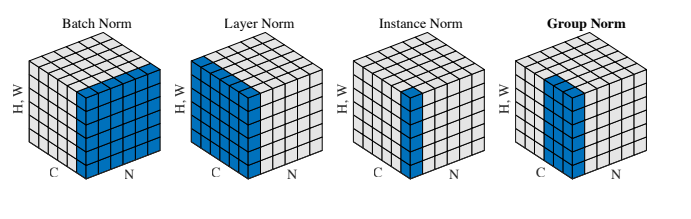## Batch Normalization

Batch Normalization (BN) 是最早出现的，也通常是效果最好的归一化方式。feature map：包含 N 个样本，每个样本通道数为 C，高为 H，宽为 W。对其求均值和方差时，将在 N、H、W上操作，而保留通道 C 的维度。具体来说，就是把第1个样本的第1个通道，加上第2个样本第1个通道 ...... 加上第 N 个样本第1个通道，求平均，得到通道 1 的均值（注意是除以 N×H×W 而不是单纯除以 N，最后得到的是一个代表这个 batch 第1个通道平均值的数字，而不是一个 H×W 的矩阵）。求通道 1 的方差也是同理。对所有通道都施加一遍这个操作，就得到了所有通道的均值和方差。具体公式为：# coding=utf8
import torch
from torch import nn

# track_running_stats=False，求当前 batch 真实平均值和标准差，
# 而不是更新全局平均值和标准差
# affine=False, 只做归一化，不乘以 gamma 加 beta（通过训练才能确定）
# num_features 为 feature map 的 channel 数目
# eps 设为 0，让官方代码和我们自己的代码结果尽量接近
bn = nn.BatchNorm2d(num_features=3, eps=0, affine=False, track_running_stats=False)

# 乘 10000 为了扩大数值，如果出现不一致，差别更明显
x = torch.rand(10, 3, 5, 5)*10000
official_bn = bn(x)

# 把 channel 维度单独提出来，而把其它需要求均值和标准差的维度融合到一起
x1 = x.permute(1,0,2,3).view(3, -1)

mu = x1.mean(dim=1).view(1,3,1,1)
# unbiased=False, 求方差时不做无偏估计（除以 N-1 而不是 N），和原始论文一致
# 个人感觉无偏估计仅仅是数学上好看，实际应用中差别不大
std = x1.std(dim=1, unbiased=False).view(1,3,1,1)

my_bn = (x-mu)/std

diff=(official_bn-my_bn).sum()
print('diff={}'.format(diff)) # 差别是 10-5 级的，证明和官方版本基本一致

## Layer Normalization

BN 的一个缺点是需要较大的 batchsize 才能合理估训练数据的均值和方差，这导致内存很可能不够用，同时它也很难应用在训练数据长度不同的 RNN 模型上。Layer Normalization (LN) 的一个优势是不需要批训练，在单条数据内部就能归一化。import torch
from torch import nn

x = torch.rand(10, 3, 5, 5)*10000

# normalization_shape 相当于告诉程序这本书有多少页，每页多少行多少列
# eps=0 排除干扰
# elementwise_affine=False 不作映射
# 这里的映射和 BN 以及下文的 IN 有区别，它是 elementwise 的 affine，
# 即 gamma 和 beta 不是 channel 维的向量，而是维度等于 normalized_shape 的矩阵
ln = nn.LayerNorm(normalized_shape=[3, 5, 5], eps=0, elementwise_affine=False)

official_ln = ln(x)

x1 = x.view(10, -1)
mu = x1.mean(dim=1).view(10, 1, 1, 1)
std = x1.std(dim=1,unbiased=False).view(10, 1, 1, 1)

my_ln = (x-mu)/std

diff = (my_ln-official_ln).sum()

print('diff={}'.format(diff)) # 差别和官方版本数量级在 1e-5

## Instance Normalization

Instance Normalization (IN) 最初用于图像的风格迁移。作者发现，在生成模型中， feature map 的各个 channel 的均值和方差会影响到最终生成图像的风格，因此可以先把图像在 channel 层面归一化，然后再用目标风格图片对应 channel 的均值和标准差“去归一化”，以期获得目标图片的风格。IN 操作也在单个样本内部进行，不依赖 batch。IN 求均值时，相当于把一页书中所有字加起来，再除以该页的总字数：H×W，即求每页书的“平均字”，求标准差时也是同理。

import torch
from torch import nn

x = torch.rand(10, 3, 5, 5) * 10000

# track_running_stats=False，求当前 batch 真实平均值和标准差，
# 而不是更新全局平均值和标准差
# affine=False, 只做归一化，不乘以 gamma 加 beta（通过训练才能确定）
# num_features 为 feature map 的 channel 数目
# eps 设为 0，让官方代码和我们自己的代码结果尽量接近
In = nn.InstanceNorm2d(num_features=3, eps=0, affine=False, track_running_stats=False)

official_in = In(x)

x1 = x.view(30, -1)
mu = x1.mean(dim=1).view(10, 3, 1, 1)
std = x1.std(dim=1, unbiased=False).view(10, 3, 1, 1)

my_in = (x-mu)/std

diff = (my_in-official_in).sum()
print('diff={}'.format(diff)) # 误差量级在 1e-5

## Group Normalization

Group Normalization (GN) 适用于占用显存比较大的任务，例如图像分割。对这类任务，可能 batchsize 只能是个位数，再大显存就不够用了。而当 batchsize 是个位数时，BN 的表现很差，因为没办法通过几个样本的数据量，来近似总体的均值和标准差。GN 也是独立于 batch 的，它是 LN 和 IN 的折中。正如提出该算法的论文展示的：

GN 计算均值和标准差时，把每一个样本 feature map 的 channel 分成 G 组，每组将有 C/G 个 channel，然后将这些 channel 中的元素求均值和标准差。各组 channel 用其对应的归一化参数独立地归一化。import torch
from torch import nn

x = torch.rand(10, 20, 5, 5)*10000

# 分成 4 个 group
# 其余设定和之前相同
gn = nn.GroupNorm(num_groups=4, num_channels=20, eps=0, affine=False)
official_gn = gn(x)

# 把同一 group 的元素融合到一起
x1 = x.view(10, 4, -1)
mu = x1.mean(dim=-1).reshape(10, 4, -1)
std = x1.std(dim=-1).reshape(10, 4, -1)

x1_norm = (x1-mu)/std
my_gn = x1_norm.reshape(10, 20, 5, 5)

diff = (my_gn-official_gn).sum()

print('diff={}'.format(diff)) # 误差在 1e-4 级

## 总结

• BN 相当于把这些书按页码一一对应地加起来（例如：第1本书第36页，加第2本书第36页......），再除以每个页码下的字符总数：N×H×W，因此可以把 BN 看成求“平均书”的操作（注意这个“平均书”每页只有一个字）
• LN 相当于把每一本书的所有字加起来，再除以这本书的字符总数：C×H×W，即求整本书的“平均字”
• IN 相当于把一页书中所有字加起来，再除以该页的总字数：H×W，即求每页书的“平均字”
• GN 相当于把一本 C 页的书平均分成 G 份，每份成为有 C/G 页的小册子，对这个 C/G 页的小册子，求每个小册子的“平均字”